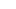# PARALLEL NUMERICAL ALGORITHMS  @VT

## ABOUT THE PARALLEL NUMERICAL ALGORITHMS RESEARCH GROUP

Tim Warburton directs the Parallel Numerical Algorithms research group @ Virginia Tech.

The majority of floating point computational performance on modern extreme scale systems is provided by massively parallel graphics processing units. For instance the latest Volta class GPU from NVIDIA has more than 5000 floating point units. The ParaNumAl research group is focused on designing algorithms for finite element analysis that fully exploit the parallelism and data movement capabilities of these GPUs.

Our research is currently funded in part through the John K. Costain Faculty Chair at VT and the DOE Center for Efficient Exascale Discretizations. More information about Professor Costain can be found here.

## LOWDOWN ON HIGH-ORDER BLOG

October 11, 2018

Ray Tracing Visualization of Sphere Constrained Flow

September 26, 20183D ellipsoids: rendering and collision detection

September 18, 20181/10

## LOW-ORDER PRECONDITIONING OF HIGH-ORDER TRIANGULAR FINITE ELEMENTS

We propose a new formulation of a low-order elliptic preconditioner for high-order triangular elements. In the preconditioner, the nodes of the low-order finite element problem do not necessarily coincide with the high-order nodes. Instead, the two spaces are connected using least squares projection operators...

## DISCONTINUOUS GALERKIN DISCRETIZATIONS OF THE BOLTZMANN EQUATIONS IN 2D: SEMI-ANALYTIC TIME STEPPING AND ABSORBING BOUNDARY LAYERS

We present an efficient nodal discontinuous Galerkin method for approximating nearly incompressible flows using the Boltzmann equations. The equations are discretized with Hermite polynomials in velocity space yielding a first order conservation law. A stabilized unsplit perfectly matching layer (PML) formulation is introduced for the resulting nonlinear flow equations. The proposed PML equations exponentially absorb the difference between the nonlinear fluctuation and the prescribed mean flow. We introduce semi-analytic time discretization methods to improve the time step restrictions in small relaxation times. We also introduce a multirate semi-analytic Adams-Bashforth method which preserves efficiency in stiff regimes. Accuracy and performance of the method are tested using distinct cases including isothermal vortex, flow around square cylinder, and wall mounted square cylinder test cases.

## AN ENTROPY STABLE DISCONTINUOUS GALERKIN METHOD FOR THE SHALLOW WATER EQUATIONS ON CURVILINEAR MESHES WITH WET/DRY FRONTS ACCELERATED BY GPUS

We extend the entropy stable high order nodal discontinuous Galerkin spectral element approximation for the non-linear two dimensional shallow water equations presented by Wintermeyer et al. [Journal of Computational Physics, 340:200-242, 2017] with a shock capturing technique and a positivity preservation capability to handle dry areas. The scheme preserves the entropy inequality, is well-balanced and works on unstructured, possibly curved, quadrilateral meshes. For the shock capturing, we introduce an artificial viscosity to the equations and prove that the numerical scheme remains entropy stable. We add a positivity preserving limiter to guarantee non-negative water heights as long as the mean water height is non-negative. We prove that non-negative mean water heights are guaranteed under a certain additional time step restriction for the entropy stable numerical interface flux. We implement the method on GPU architectures using the abstract language OCCA, a unified approach to multi-threading languages. We show that the entropy stable scheme is well suited to GPUs as the necessary extra calculations do not negatively impact the runtime up to reasonably high polynomial degrees (around N=7). We provide numerical examples that challenge the shock capturing and positivity properties of our scheme to verify our theoretical findings.

## GPU ACCELERATION OF A HIGH-ORDER DISCONTINUOUS GALERKIN INCOMPRESSIBLE FLOW SOLVER

We present a GPU-accelerated version of a high-order discontinuous Galerkin discretization of the unsteady incompressible Navier-Stokes equations. The equations are discretized in time using a semi-implicit scheme with explicit treatment of the nonlinear term and implicit treatment of the split Stokes operators. The pressure system is solved with a conjugate gradient method together with a fully GPU-accelerated multigrid preconditioner which is designed to minimize memory requirements and to increase overall performance. A semi-Lagrangian subcycling advection algorithm is used to shift the computational load per timestep away from the pressure Poisson solve by allowing larger timestep sizes in exchange for an increased number of advection steps. Numerical results confirm we achieve the design order accuracy in time and space. We optimize the performance of the most time-consuming kernels by tuning the fine-grain parallelism, memory utilization, and maximizing bandwidth. To assess overall performance we present an empirically calibrated roofline performance model for a target GPU to explain the achieved efficiency. We demonstrate that, in the most cases, the kernels used in the solver are close to their empirically predicted roofline performance.

## ACCELERATION OF TENSOR-PRODUCT OPERATIONS FOR HIGH-ORDER FINITE ELEMENT METHODS

This paper is devoted to GPU kernel optimization and performance analysis of three tensor-product operators arising in finite element methods. We provide a mathematical background to these operations and implementation details. Achieving close-to-the-peak performance for these operators requires extensive optimization because of the operators' properties: low arithmetic intensity, tiered structure, and the need to store intermediate results inside the kernel. We give a guided overview of optimization strategies and we present a performance model that allows us to compare the efficacy of these optimizations against an empirically calibrated roofline.

## GET IN TOUCH

225 Stanger St
Blacksburg, VA 24061
USA.

225 Stanger St
Blacksburg, VA 24061
USA.

©2018 BY THE PARALLEL NUMERICAL ALGORITHMS  RESEARCH GROUP @VT.